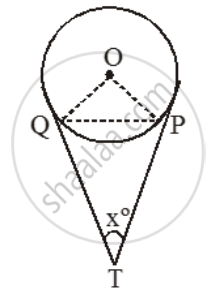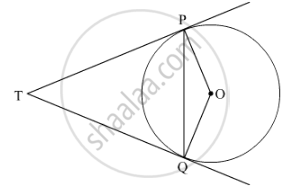# Two tangents TP and TQ are drawn to a circle with centre O from an external point T. Prove that ∠PTQ = 2∠OPQ. - Mathematics

Sum

Two tangents TP and TQ are drawn to a circle with centre O from an external point T. Prove that ∠PTQ = 2∠OPQ.

#### Solution 1

Given: A circle with centre O and an external point T from which tangents TP and TQ are drawn to touch the circle at P and Q.To prove: ∠PTQ = 2∠OPQ.

Proof: Let ∠PTQ = xº. Then,

∠TQP + ∠TPQ + ∠PTQ = 180º

[∵ sum of the ∠s of a triangle is 180º]

⇒ ∠TQP + ∠TPQ = (180º – x) ….(i)

We know that the lengths of tangent drawn from an external point to a circle are equal.

So, TP = TQ.

Now, TP = TQ

⇒ ∠TQP = ∠TPQ

=\frac{1}{2}(180^{0}-x)=( 90^\text{o}-\frac{x}{2})

= (180º –x)=

∴ ∠OPQ = (∠OPT–∠TPQ)

=90^{0}-( 90^\text{o}-\frac{x}{2})=\frac{x}{2}

⇒ ∠OPQ = \frac { 1 }{ 2 } ∠PTQ

⇒ ∠PTQ = 2∠OPQ

#### Solution 2Given: TP and TQ are two tangents of a circle with centre O and P and Q are points of contact

To prove: ∠PTQ = 2∠OPQ

Suppose ∠PTQ = θ.

Now by theorem, "The lengths of a tangents drawn from an external point to a circle are equal".

So, TPQ is an isoceles triangle.

Therefore, ∠TPQ = ∠TQP = 1/2(180°−θ)=90°−θ/2.

Also by theorem "The tangents at any point of a circle is perpendicular to the radius through the point of contact" ∠OPT=90°.

Therefore,

∠OPQ=∠OPT−∠TPQ

= 90^@ - (90^@ -  1/2theta)

= 1/2 theta

= 1/2 PTQ

Hence, ∠PTQ = 2∠OPQ.

Concept: Concept of Circle - Centre, Radius, Diameter, Arc, Sector, Chord, Segment, Semicircle, Circumference, Interior and Exterior, Concentric Circles
Is there an error in this question or solution?